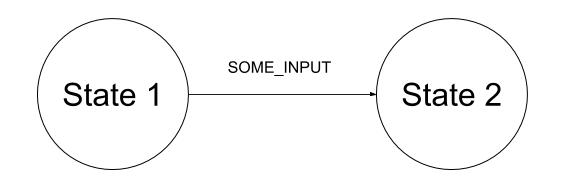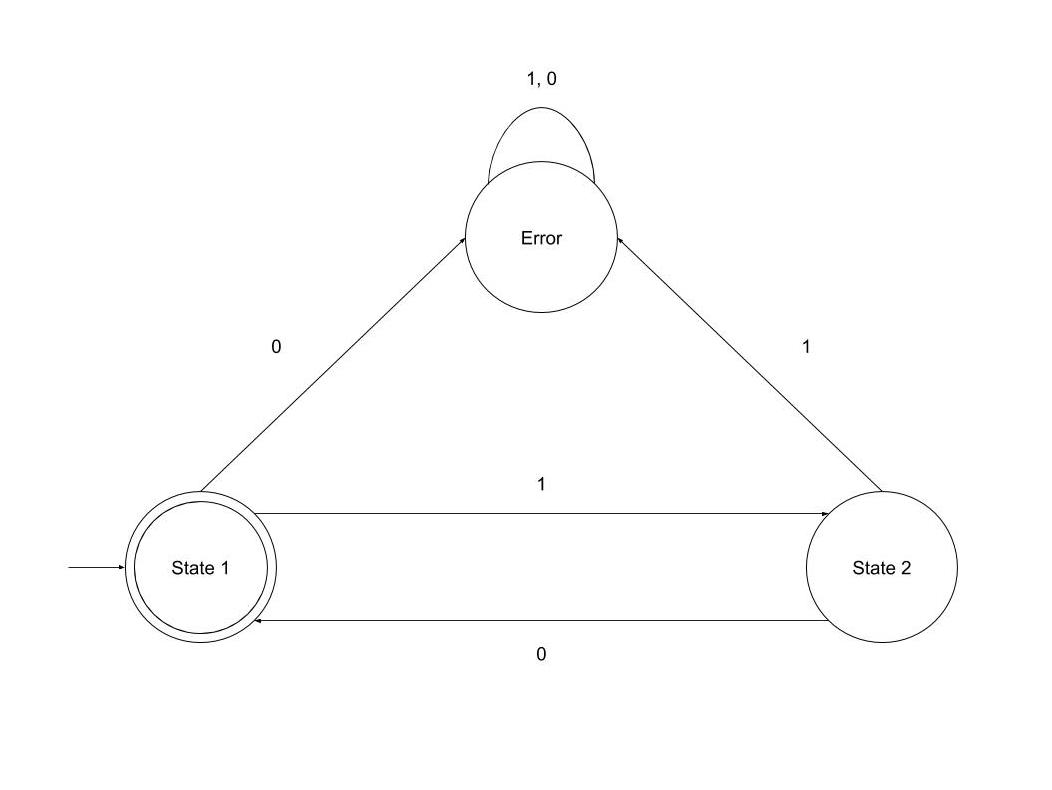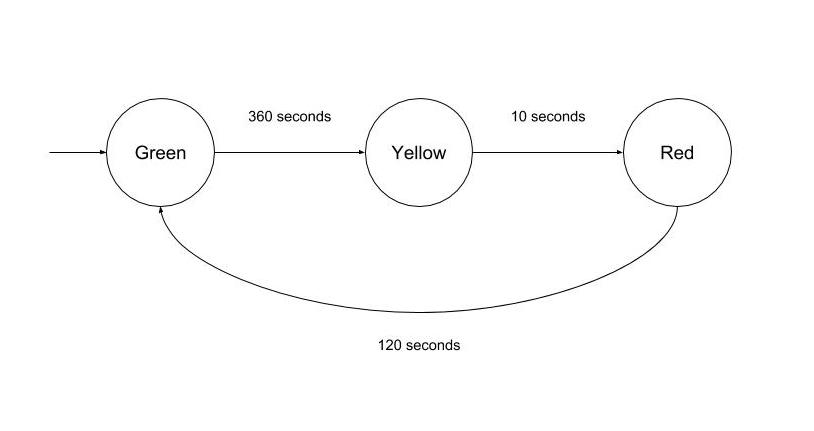Theory of Computation: Finite State Machines - Stack Abuse

# Theory of Computation: Finite State Machines### Introduction

A Finite State Machine is a model of computation, i.e. a conceptual tool to design systems. It processes a sequence of inputs that changes the state of the system.

When all the input is processed, we observe the system's final state to determine whether the input sequence was accepted or not.

### Finite State Machine Components

Finite State Machines are comprised of these components:

• Set of Known States: as the name implies, there must be a finite amount of states our system can be in. Only one state can be active at a time. States are usually drawn with circles:• Initial State: the starting point of our system. Initial states are usually drawn with an arrow being pointed to them:• Set of Accepting States: a subset of known states that indicates whether the input we processed is valid or not. Accepting states are usually drawn as a double circle:• Alphabet: also referred to as Language, is the set of all valid inputs.
• Transitions: rules dictating how the machine moves from one state to another. These are usually drawn as two states connected by a line:### Binary Parser Demonstration

Let's create a Finite State Machine that parses a binary sequence where every time we encounter a 1, we immediately encounter a 0. For example, 1010 is a valid input sequence but 1110 and 1010100 are not.

We can create a Finite State Machine like this:Our Finite State Machine has 3 states: State 1, State 2 and Error.

Here, we have a few possible situations:

• If we're at State 1 and encounter a 1, we move to State 2.
• If we're at State 2 and encounter a 0, we move back to State 1.
• If we're at State 1 and encounter a 0, we go to the Error state.
• If we're at State 2 and encounter a 1, we go to the Error state.

As you would observe, any input in the Error state keeps it there, as you are not able to transition out of the error state.

### Creating an FSM in Python

Many software solutions implement Finite State Machines to manage some aspect of states. We can implement a Finite State Machine in Python and programmatically verify input for a given set of states and transitions:

class Transition:
"""A change from one state to a next"""

def __init__(self, current_state, state_input, next_state):
self.current_state = current_state
self.state_input = state_input
self.next_state = next_state

def match(self, current_state, state_input):
"""Determines if the state and the input satisfies this transition relation"""
return self.current_state == current_state and self.state_input == state_input

class FSM:
"""A basic model of computation"""

def __init__(
self,
states=[],
alphabet=[],
accepting_states=[],
initial_state=''):
self.states = states
self.alphabet = alphabet
self.accepting_states = accepting_states
self.initial_state = initial_state
self.valid_transitions = False

def add_transitions(self, transitions=[]):
"""Before we use a list of transitions, verify they only apply to our states"""
for transition in transitions:
if transition.current_state not in self.states:
print(
'Invalid transition. {} is not a valid state'.format(
transition.current_state))
return
if transition.next_state not in self.states:
print('Invalid transition. {} is not a valid state'.format)
return
self.transitions = transitions
self.valid_transitions = True

def __accept(self, current_state, state_input):
"""Looks to see if the input for the given state matches a transition rule"""
# If the input is valid in our alphabet
if state_input in self.alphabet:
for rule in self.transitions:
if rule.match(current_state, state_input):
return rule.next_state
print('No transition for state and input')
return None
return None

def accepts(self, sequence):
"""Process an input stream to determine if the FSM will accept it"""
# Check if we have transitions
if not self.valid_transitions:
print('Cannot process sequence without valid transitions')

print('Starting at {}'.format(self.initial_state))
# When an empty sequence provided, we simply check if the initial state
# is an accepted one
if len(sequence) == 0:
return self.initial_state in self.accepting_states

# Let's process the initial state
current_state = self.__accept(self.initial_state, sequence)
if current_state is None:
return False

# Continue with the rest of the sequence
for state_input in sequence[1:]:
print('Current state is {}'.format(current_state))
current_state = self.__accept(current_state, state_input)
if current_state is None:
return False

print('Ending state is {}'.format(current_state))
# Check if the state we've transitioned to is an accepting state
return current_state in self.accepting_states


## Better understand your data with visualizations

•  30-day no-questions refunds
•  Beginner to Advanced
•  Updated regularly (update June 2021)
•  New bonus resources and guides

The Transition class contains a match() function. A quick way for us to know if the current state and input of the Finite State Machine will allow us to go to the next state defined.

The FSM class, after being initialized, needs the add_transitions() method to be called. This method validates that our transition rules are set up with valid states.

We can then use the accepts() method with a list of inputs to determine if our machine would be in an accepting state.

Let's test it out with the binary parser we created previously. Follow the code, run it, and take note of the results:

# Set up our FSM with the required info:
# Set of states
states = ['State 1', 'State 2', 'Error']
# Set of allowed inputs
alphabet = [1, 0]
# Set of accepted states
accepting_states = ['State 1']
# The initial state
initial_state = 'State 1'
fsm = FSM(states, alphabet, accepting_states, initial_state)

# Create the set of transitions
transition1 = Transition('State 1', 1, 'State 2')
transition2 = Transition('State 2', 0, 'State 1')
transition3 = Transition('State 1', 0, 'Error')
transition4 = Transition('State 2', 1, 'Error')
transition5 = Transition('Error', 1, 'Error')
transition6 = Transition('Error', 0, 'Error')
transitions = [
transition1,
transition2,
transition3,
transition4,
transition5,
transition6]
# Verify and add them to the FSM
fsm.add_transitions(transitions)

# Now that our FSM is correctly set up, we can give it input to process
# Let's transition the FSM to a green light
should_be_accepted = fsm.accepts([1, 0, 1, 0])
print(should_be_accepted)

# Let's transition the FSM to a red light
should_be_rejected_1 = fsm.accepts([1, 1, 1, 0])
print(should_be_rejected_1)

# Let's transition to yellow and give it bad input
should_be_rejected_2 = fsm.accepts([1, 0, 1, 0, 1, 0, 0])
print(should_be_rejected_2)


### Examples

Finite State Machines are commonly used in real world systems that extend beyond string parsing, and even beyond software systems.

#### Traffic lights

A simple traffic light system can be modeled with a Finite State Machine. Let's look at each core component and identify what it would be for traffics lights:

• States: a traffic light generally has three states: Red, Green and Yellow.
• Initial State: Green
• Accepting States: in the real world traffic lights run indefinitely, so there would be no accepting states for this model.
• Alphabet: positive integers representing seconds.
• Transitions:
• If we're in state Green and wait for 360 seconds (6 minutes), then we can go to state Yellow.
• If we're in state Yellow and wait 10 seconds, then we can go to state Red.
• If we're in state Red and wait 120 seconds, then we can go to state Green.

This Finite State Machine can be drawn as follows:#### Enemy AI

Finite State Machines allows us to map the flow of actions in a game's computer-controlled players. Let's say we were making an action game where guards patrol an area of the map. We can have a Finite State Machine with the following properties:

• States: For our simplistic shooter we can have: Patrol, Attack, Reload, Take Cover, and Deceased.
• Initial State: As it's a guard, the initial state would be Patrol.
• Accepting States: An enemy bot can no longer accept input when it's dead, so our Deceased state will be our accepting one.
• Alphabet: For simplicity, we can use string constants to represent a world state: Player approaches, Player runs, Full health, Low health, No health, Full ammo, and Low ammo.
• Transitions: As this model is a bit more complex than traffic lights, we can separate the transitions by examining one state at a time:
• Patrol
• If a player approaches, go to the Attack state.
• If we run out of health, go to the Deceased state.
• Attack
• If ammo is low, go to the Reload state.
• If health is low, go to the Take Cover state.
• If the player escapes, go to the Patrol state.
• If we run out of health, go to the Deceased state.
• Reload
• If ammo is full, go to the Attack state.
• If health is low, go to the Take Cover state.
• If we run out of health, go to the Deceased state.
• Take Cover
• If health is full, go to the Attack state.
• If ammo is low, go to the Reload state.
• If we run out of health, go to the Deceased state.

This Finite State Machine can be drawn as follows:### Limitations

The primary benefit of Finite State Machines, their simplicity, also becomes one of their disadvantages. Systems which need an indeterminate amount of states cannot be modeled by a Finite State Machine, evidently.

Earlier we modeled a Finite State Machine that accepted the string '10' for any amount of iterations. If we had to accept a string of any number of 1s followed by the same number of 0s, we cannot create a Finite State Machine for it. A Finite State Machine does not keep track of the number of states it visited, it is only aware of the current state it is in.

This is proven by the Pumping Lemma, a proof which asserts that if a language is not regular (not so much Regular Expressions, which you may be familiar with from programming languages, but rather a classification of languages then no Finite State Machine can be built for it. You can learn more about this proof and its implications here.

### Conclusion

Finite State Machines are a theoretical framework we can use to model systems. Given a known set of states, the starting state, the accepting state and the rules to transition between states, we can determine if a sequence of inputs would be accepted.

The limited amount of states that can be modeled does not make these abstract machines suitable for all systems, as shown by the Pumping Lemma. Even so, Finite State Machines have many real-world applications and are popular because of their simplicity.

Last Updated: March 3rd, 2019

## Improve your dev skills!

Get tutorials, guides, and dev jobs in your inbox.

No spam ever. Unsubscribe at any time. Read our Privacy Policy.

Marcus SanatanAuthor

Web Dev|Games|Music|Art|Fun|Caribbean

I love many things and coding is one of them!

# Prepping for an interview?

• Improve your skills by solving one coding problem every day
• Get the solutions the next morning via email
• Practice on actual problems asked by top companies, like:

# Better understand your data with visualizations

•  30-day no-questions refunds
•  Beginner to Advanced
•  Updated regularly (update June 2021)
•  New bonus resources and guides

© 2013-2021 Stack Abuse. All rights reserved.